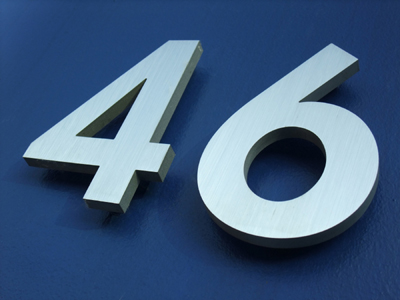If 6 - 5 = 1, then 46 - 5 = 41.

# Year 2 Calculation - Addition and Subtraction of One-Digit Numbers

This quiz addresses the requirements of the National Curriculum KS1 Maths and Numeracy for children aged 6 and 7 in year 2. Specifically this quiz is aimed at the section dealing with addition and subtraction of one digit numbers from two digit numbers.

Adding and subtracting one digit numbers from a two digit number follows on closely from the skill of adding and subtracting using only one digit numbers. At first, this calculating may rely on using equipment or fingers to check an answer, but will gradually progress to using only a number line and then perhaps visualising that line mentally.

Question 1
What is 5 + 32?
73
325
532
37
Again, changing the numbers round makes the calculation easier, and still gives the same answer
Question 2
What is 46 - 9?
37
56
36
29
Taking away 10 and then adding back a 1 is useful when subtracting 9
Question 3
What is 51 + 8?
59
60
68
54
Changing the units in the calculation to 58 + 1 might make it easier, and still gives the same answer
Question 4
What is 12 - 7?
5
6
4
19
Counting up from 7 to 12 would give the same answer as counting back from 12 to 7
Question 5
What is 42 + 8?
52
48
44
50
Knowing number bonds may help to recognise that 2 + 8 = 10
Question 6
What is 4 + 20?
42
24
204
420
This addition could be done in a different order to make it easier: 20 + 4
Question 7
What is 20 - 8?
2
18
22
12
Knowing number bonds to 20 is useful for subtractions such as this
Question 8
What is 34 - 5?
39
27
29
30
Try to picture this calculation as 34 - 4 - 1 to make it easier to calculate mentally
Question 9
What is 60 - 8?
62
58
52
68
Knowing number bonds well is useful in all sorts of calculations
Question 10
What is 55 - 7?
63
47
48
50
Counting back in two jumps (of 5 and 2) makes this calculation easier